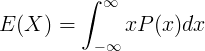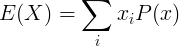# Expectation Value

In probability and statistics, the expectation or expected value, is the weighted average value of a random variable.

### Expectation of continuous random variableE(X) is the expectation value of the continuous random variable X

x is the value of the continuous random variable X

P(x) is the probability density function

### Expectation of discrete random variableE(X) is the expectation value of the continuous random variable X

x is the value of the continuous random variable X

P(x) is the probability mass function of X

### Properties of expectation

##### Linearity

When a is constant and X,Y are random variables:

E(aX) = aE(X)

E(X+Y) = E(X) + E(Y)

##### Constant

When c is constant:

E(c) = c

##### Product

When X and Y are independent random variables:

E(X ⋅Y) = E(X) ⋅ E(Y)